#### The cost of a plan

The next task is to extend the definition of the cost-to-go under a fixed plan, which was given in Section 10.1.3, to the case of imperfect state information. Consider evaluating the quality of a plan, so that the best'' one might be selected. Suppose that the nondeterministic uncertainty is used to model nature and that a nondeterministic initial condition is given. If a plan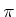is fixed, some state and action trajectories are possible, and others are not. It is impossible to know in general what histories will occur; however, the plan constrains the choices substantially. Let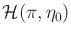denote the set of state-action histories that could arise fromapplied to the initial condition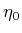.

The cost of a planfrom an initial conditionis measured using worst-case analysis as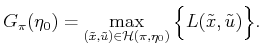(11.22)

Note that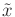includes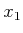, which is usually not known. It may be known only to lie in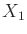, as specified by. Let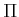denote the set of all possible plans. An optimal plan using worst-case analysis is any plan for which (11.22) is minimized over all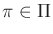and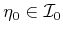. In the case of feasible planning, there are usually numerous equivalent alternatives.

Under probabilistic uncertainty, the cost of a plan can be measured using expected-case analysis as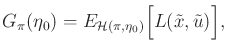(11.23)

in which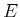denotes the mathematical expectation of the cost, with the probability distribution taken over. The task is to find a planthat minimizes (11.23).

Steven M LaValle 2020-08-14